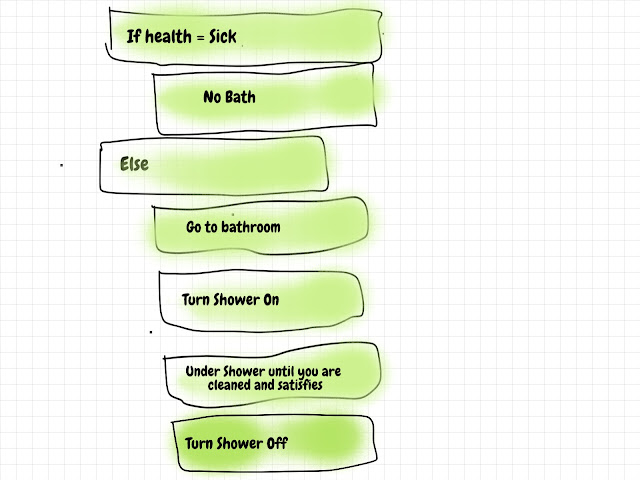### What is an algorithm?

In this tutorial, we will learn basic concepts of algorithms and their application. We will also learn about Pseudo Code.

## Introduction

The algorithm is not a concept unique to computation. It is everywhere. Even we use it knowingly or unknowingly, to carry out day-to-day activities or solve specific problems.

### Taking bath algorithm

Taking bath is a daily activity and we follow certain steps to carry out it.Taking Bath Algorithm

### Car parking algorithm

We are writing a car parking algorithm to park a car at a parking spot. All numbers are hypothetical.

Step 1: Go to the parking lot using the main gate.

Step 2: Go straight for 10 meters.

Step 3: Take a left turn.

Step 4: Go straight for 8 meters.

Step 5: Turn the car at 45-degree angle.

Step 6: Move back your car at the same angle for 12 meters

Step 7: Stop Engine Ignition.

Step 8: Lock car gates.

### Algorithmic thinking

If we write down the steps required to carry out our day-to-day activities or problem solving. We came across the following computational concepts:

1. Loop or Repetition - Repeating certain steps again and again. For e.g. Sprinkle water on the plant 10 times (for 10 plants of course).
2. Sequencing - Following one step after another or so on. For e.g. Wearing shoes require the following steps in a sequence: pull a shoe from the shoe rack, putting on a sock, and wearing shoes. Not following steps in sequence will lead to a problem.
3. Conditional Logic - Out of two possible steps which one to follow. For e.g. If it is Friday I will wear casual otherwise formal clothes.

When we break down any activity into discrete steps and create an algorithm like we did before, this way of thinking is called algorithmic thinking. It is a powerful tool for better decision-making in life because it brings clarity to our actions.

## What is an algorithm in computation?

Our expectation from computers is to help in solving real life problems with fast and accurate results in comparison to manual work. The algorithm is one of the most important concepts of computer science. It provides tools and methodologies to solve well-defined computational problems.

The algorithm is an idea and a plan to solve well-defined general-purpose computational problems. It takes Input, processes them using step-by-step instruction, and produces the expected output.

For e.g. Finding the max value in a list of numbers.

Pseudo Code:

``````SET Max to listOfNumbers\[0\]
FOR i = 1 to listOfNumbers length - 1
IF listOfNumbers\[i\] > Max THEN
SET Max to listOfNumbers\[i\]
ENDIF
ENDFOR
PRINT Max
``````

It is a well-defined general purpose problem that clearly mentions the Input and expected output. It will work for any Input consisting it is a list of numbers.

If a problem is not well-defined and general purpose, we are not finding an algorithm but solving a very specific problem, which may or may not an algorithm for a general purpose problem.

We evaluate the algorithm against these important factors:

1. Correctness - An algorithm need to yield the correct result for all possible inputs.
2. Efficiency - What is the performance of an algorithm against the size of inputs.

Another important factor is implementation complexity, but it is not as significant as correctness and efficiency. But in situations when we have two algorithms with similar efficiency, we will select which is easier to implement.

## PseudoCode

We can represent an algorithm using plain English, pseudo code, flow chart (for small problems) or program.

Plain English is very descriptive while programs are very complex to read. Pseudo Code is easier to read while looking like a program without any syntax validation.

Note: Pseudocode is not a standard but it's a convention.

### Pseudocode notations

Most common Pseudocode notations:

1. INPUT – To take user input.
2. SET - Set value in a variable.
3. INITIALIZE - initialize a variable with a value.
4. WHILE – A loop with a condition at the beginning.
5. FOR – A loop with a counter.
6. REPEAT – UNTIL – A loop with the condition at the end.
7. IF – THEN – ELSE – It denotes conditional logic or decision-making for multiple choices.
8. OUTPUT – To display output on-screen, print, file, etc.
9. PRINT - Print output.

Important points:

1. Indentation - Any steps that occur inside a choice or iteration is usually indented.
2. Convention not rule - You are free to make changes to the convention.
3. The capital case for notations - You may use lowercase or CamelCase whatever suits you.

### Pseudocode examples

#### Example 1: Print Pass or Fail for a score

Input: A number

Output: If the input number is 50 or more than Output Pass otherwise fail

Pseudocode

``````INPUT score
IF score >= 50
OUTPUT Pass
ELSE
OUTPUT Fail
``````

#### Example 2: Average classroom scores

Input: A list of numbers

Output: A number representing an average of the input list of numbers

Pseudocode

``````INPUT scores into listOfScore
SET total to 0
SET noOfScore to 0
SET classAverage to 0

WHILE length of listOfScore > noOfScore
SET total = total + listOfScore\[noOfScore\]
SET noOfScore = noOfScore + 1

SET classAverage = total divided by noOfScore
OUTPUT classAverage
``````